Next: The Gaussian distribution Up: Probability theory Previous: The mean, variance, and

## Application to the binomial distribution

Let us now apply what we have just learned about the mean, variance, and standard deviation of a general distribution function to the specific case of the binomial distribution function. Recall, that if a simple system has just two possible outcomes, denoted 1 and 2, with respective probabilities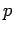and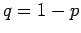, then the probability of obtaining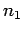occurrences of outcome 1 inobservations is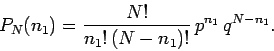(38)

Thus, the mean number of occurrences of outcome 1 inobservations is given by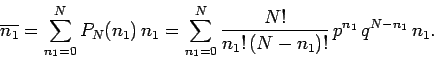(39)

This is a rather nasty looking expression! However, we can see that if the final factorwere absent, it would just reduce to the binomial expansion, which we know how to sum. We can take advantage of this fact by using a rather elegant mathematical sleight of hand. Observe that since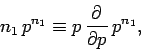(40)

the summation can be rewritten as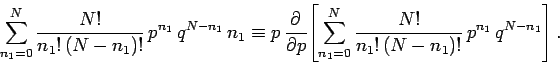(41)

This is just algebra, and has nothing to do with probability theory. The term in square brackets is the familiar binomial expansion, and can be written more succinctly as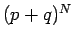. Thus,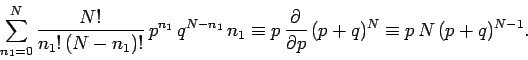(42)

However,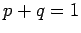for the case in hand, so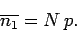(43)

In fact, we could have guessed this result. By definition, the probabilityis the number of occurrences of the outcome 1 divided by the number of trials, in the limit as the number of trials goes to infinity: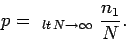(44)

If we think carefully, however, we can see that taking the limit as the number of trials goes to infinity is equivalent to taking the mean value, so that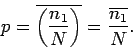(45)

But, this is just a simple rearrangement of Eq. (43).

Let us now calculate the variance of. Recall that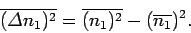(46)

We already know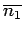, so we just need to calculate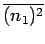. This average is written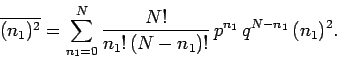(47)

The sum can be evaluated using a simple extension of the mathematical trick we used earlier to evaluate. Since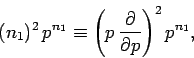(48)

then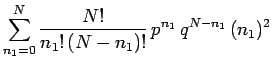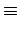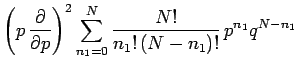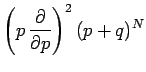(49)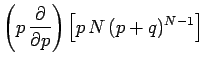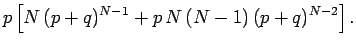Usingyields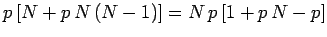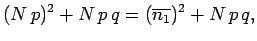(50)

since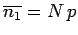. It follows that the variance ofis given by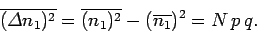(51)

The standard deviation ofis just the square root of the variance, so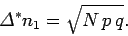(52)

Recall that this quantity is essentially the width of the range over whichis distributed around its mean value. The relative width of the distribution is characterized by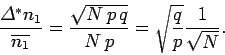(53)

It is clear from this formula that the relative width decreases like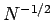with increasing. So, the greater the number of trials, the more likely it is that an observation ofwill yield a result which is relatively close to the mean value. This is a very important result.Next: The Gaussian distribution Up: Probability theory Previous: The mean, variance, and
Richard Fitzpatrick 2006-02-02